# Crystal surface analysis

## [Calculation overview]

Analysis of crystal surface is essential to understand phenomena of a solid such as dissolution, sublimation, melting and so on. CONFLEX can calculate a sum of interatomic interaction energies with respect to a molecule in or on a specified crystal plane and evaluate a stability of its plane.

For example, if you specify the (100) plane, CONFLEX builds a hemi-spherical crystal in which the (100) plane is exposed (refer to the below figure) and calculate the sum of interatomic interaction energies with respect to the molecule in or on the (100) plane. A radius of the hemi-spherical crystal is cutoff value.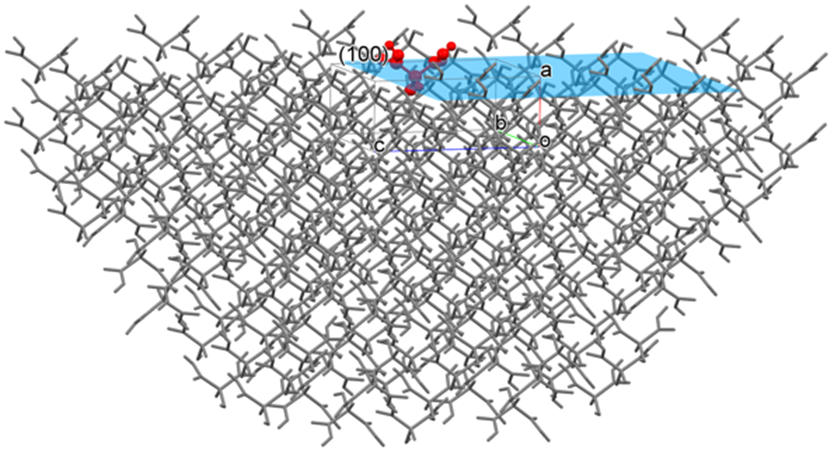Here, Emol is the sum of interatomic interaction energies with respect to the molecule (target molecule, the molecule shown in red in the above figure) in or on the center of (100) plane, N is total the number of atoms of the target molecule, and M is total the number of atoms of the hemi-spherical crystal without the target molecule.

## [How to perform the calculation]

We use a crystal of hydroxy malonic acid which is one of malonic acid derivatives [Roelofsen, G.; Kanters, J.A.; Kroon, J.; Doesburg, H.M.; Koops, T. Acta Cryst.1978, B34, 2565.].
Input file (tartronicacid.cmf) is below. You can find the tartronicacid.cmf in Sample_Files folder in the folder installed CONFLEX (Sample_Files\CONFLEX\crystal\plane\in_plane\tartronicacid.cmf).

tartronicacid.cmf filfe

data_Tartronicacid
_symmetry_cell_setting ORTHORHOMBIC
_symmetry_space_group_name_H-M 'P212121 '
_ccdc_symmetry_space_group_name P212121
_symmetry_Int_Tables_number 19
loop_
_symmetry_equiv_pos_site_id
_symmetry_equiv_pos_as_xyz
1 x,y,z
2 1/2+x,1/2-y,-z
3 -x,1/2+y,1/2-z
4 1/2-x,-y,1/2+z
_cell_length_a 4.49400
_cell_length_b 8.81900
_cell_length_c 10.88200
_cell_angle_alpha 90.00000
_cell_angle_beta 90.00000
_cell_angle_gamma 90.00000
_cell_formula_units_Z 4
_cell_volume 431.28180
_exptl_crystal_density_diffrn 1.84821
loop_
_ccdc_atom_site_atom_id_number
_atom_site_label
_atom_site_type_symbol
_atom_site_fract_x
_atom_site_fract_y
_atom_site_fract_z
_ccdc_atom_site_symmetry
_ccdc_atom_site_base
1 O1 O 1.12990 -0.13910 0.36040 1_555 1
2 O2 O 0.97510 0.09280 0.30700 1_555 2
3 O3 O 1.01480 0.11550 0.66290 1_555 3
4 O4 O 1.13030 -0.12820 0.62810 1_555 4
5 O5 O 0.57240 0.09790 0.48970 1_555 5
6 C1 C 0.97750 -0.01540 0.37600 1_555 6
7 C2 C 0.78810 -0.01520 0.49230 1_555 7
8 C3 C 0.99010 -0.00160 0.60420 1_555 8
9 H1 H 0.66800 -0.11200 0.49600 1_555 9
10 H2 H 0.60500 0.14900 0.45600 1_555 10
11 H3 H 1.23700 -0.13800 0.31000 1_555 11
12 H4 H 1.27100 -0.12000 0.68000 1_555 12
loop_
_atom_id
_atom_type
_atom_attach_nh
_atom_attach_h
_atom_charge
1 O 1 1 0
2 O 1 0 0
3 O 1 0 0
4 O 1 1 0
5 O 1 1 0
6 C 3 0 0
7 C 3 1 0
8 C 3 0 0
loop_
_bond_id_1
_bond_id_2
_bond_type_ccdc
_bond_environment
1 6 S chain
1 11 S chain
2 6 D chain
3 8 D chain
4 8 S chain
4 12 S chain
5 7 S chain
5 10 S chain
6 7 S chain
7 8 S chain
7 9 S chain

### [Execution by Interface]

Open the tartronicacid.cmf file by CONFLEX Interface.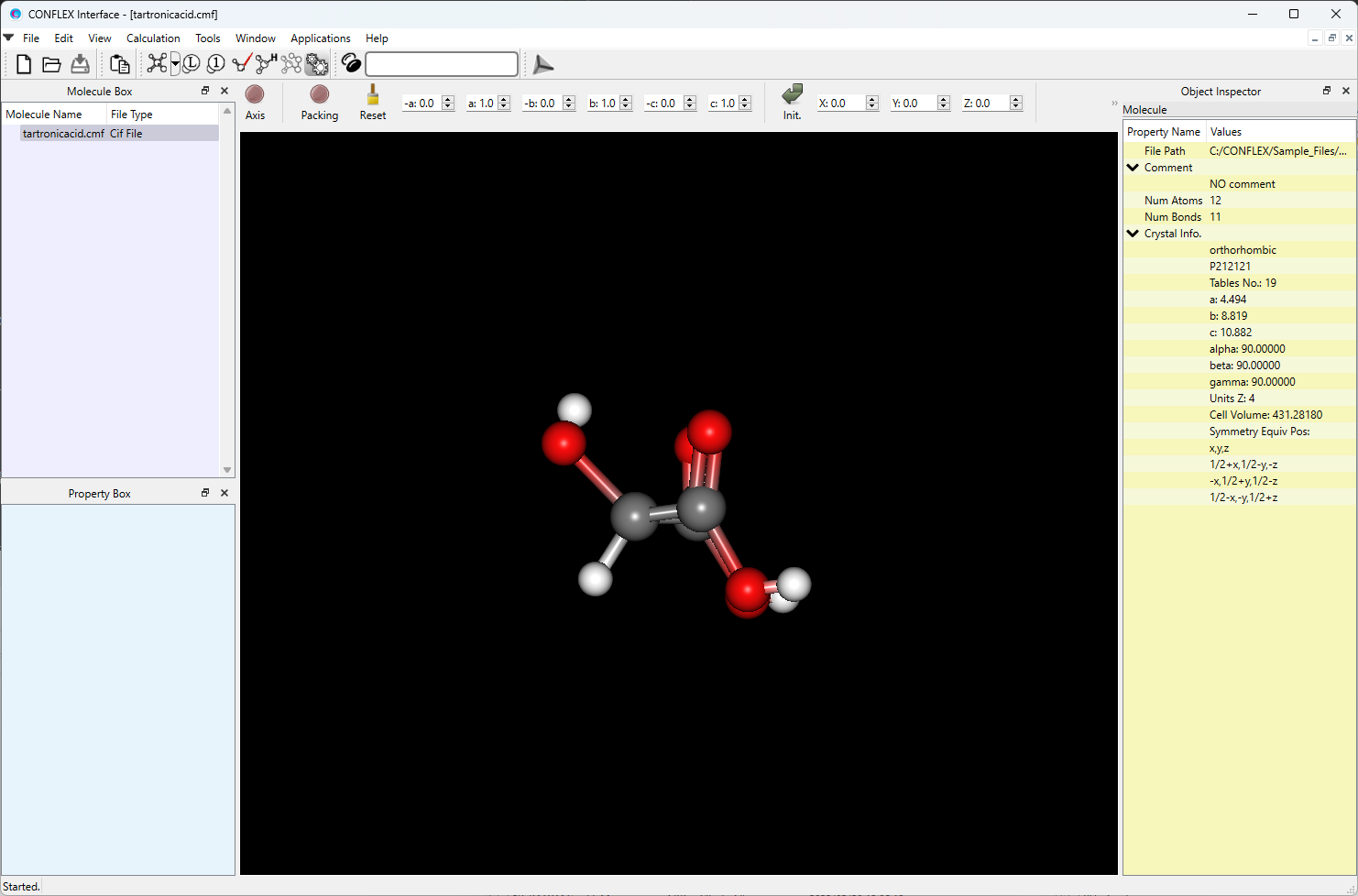Select [CONFLEX] in Calculation menu, and click in the calculation setting dialog displayed. A detail setting dialog will be displayed.First, in [General Settings] dialog on the detail setting dialog, select [Molecular Crystal] in the pull-down menu of [Calculation Type:].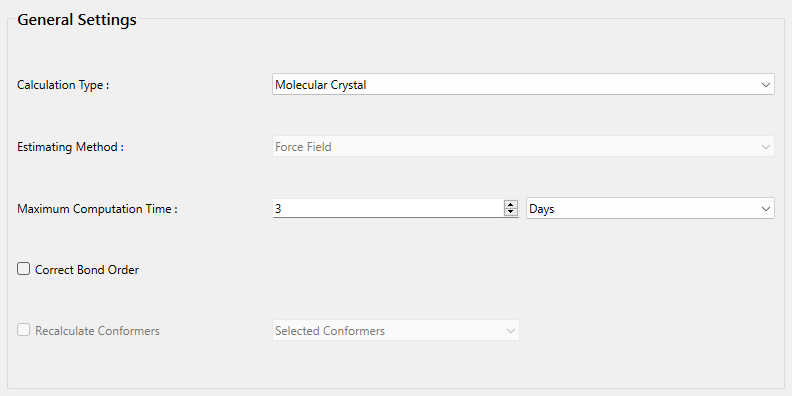Next, select [None] in the pull-down menu of [Crystal optimization:] on [Crystal Calculation] dialog. If you optimize the crystal structure before the surface analysis, you should select [ALL] or others.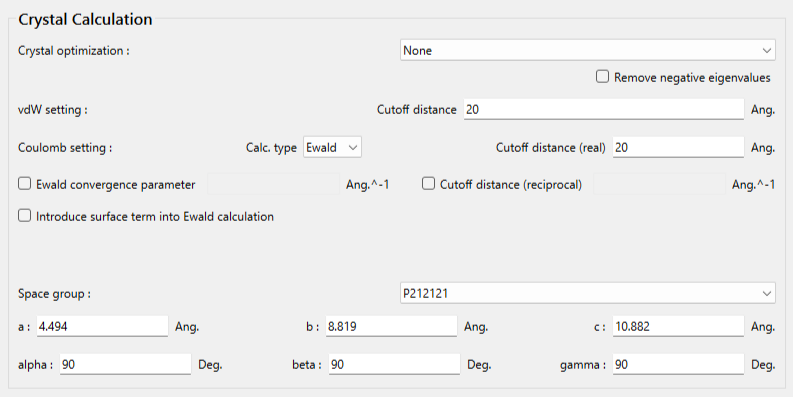Next, we perform settings for the surface analysis by [Crystal Surface] tab in [Crystal Property] dialog.First, check the check box of [Crystal Surface Analysis].
Next, set hkl indices for the crystal plane to [Miller Indices]. Here, h, k, and l set to 1, 0, and 0, respectively.
[In] of [Molecular State:] means that the Emol with respect to the molecule composing of the (100) plane is calculated.
When the calculation settings are complete, click . The calculation will start.

### [Execution by command line]

The calculation settings are defined by describing keywords in the tartronicacid.ini file.

tartronicacid.ini file

CRYSTAL
CRYSTAL_OPTIMIZATION=NONE
CRYSTAL_PLANE=(1,0,0)

[CRYSTAL] means to execute a crystal calculation.
[CRYSTAL_OPTIMIZATION=NONE] means a crystal structure optimization is not performed. If you optimize the crystal structure before the analysis, you should select [ALL] or others.
[CRYSTAL_PLANE=(1,0,0)] means an analysis for the (100) plane is performed. In default setting, the Emol with respect to the molecule composing of the (100) plane is calculated.

Store the two files of tartronicacid.cmf and tartronicacid.ini in an one folder, and execute below command. The calculation will start.

C:\CONFLEX\bin\flex9a_win_x64.exe   -par   C:\CONFLEX\par   tartronicacidenter

The above command is for Windows OS. For the other OS, please refer to [How to execute CONFLEX].

## [Calculation results]

After the calculation, you can find tartronicacid_100.pdb file. This file has structure data of the hemi-spherical with the (100) plane. The file name format is [(input file name)_hkl.pdb]. If you open the tartronicacid_100.pdb file by CONFLEX Interface, you can see the hemi-spherical crystal.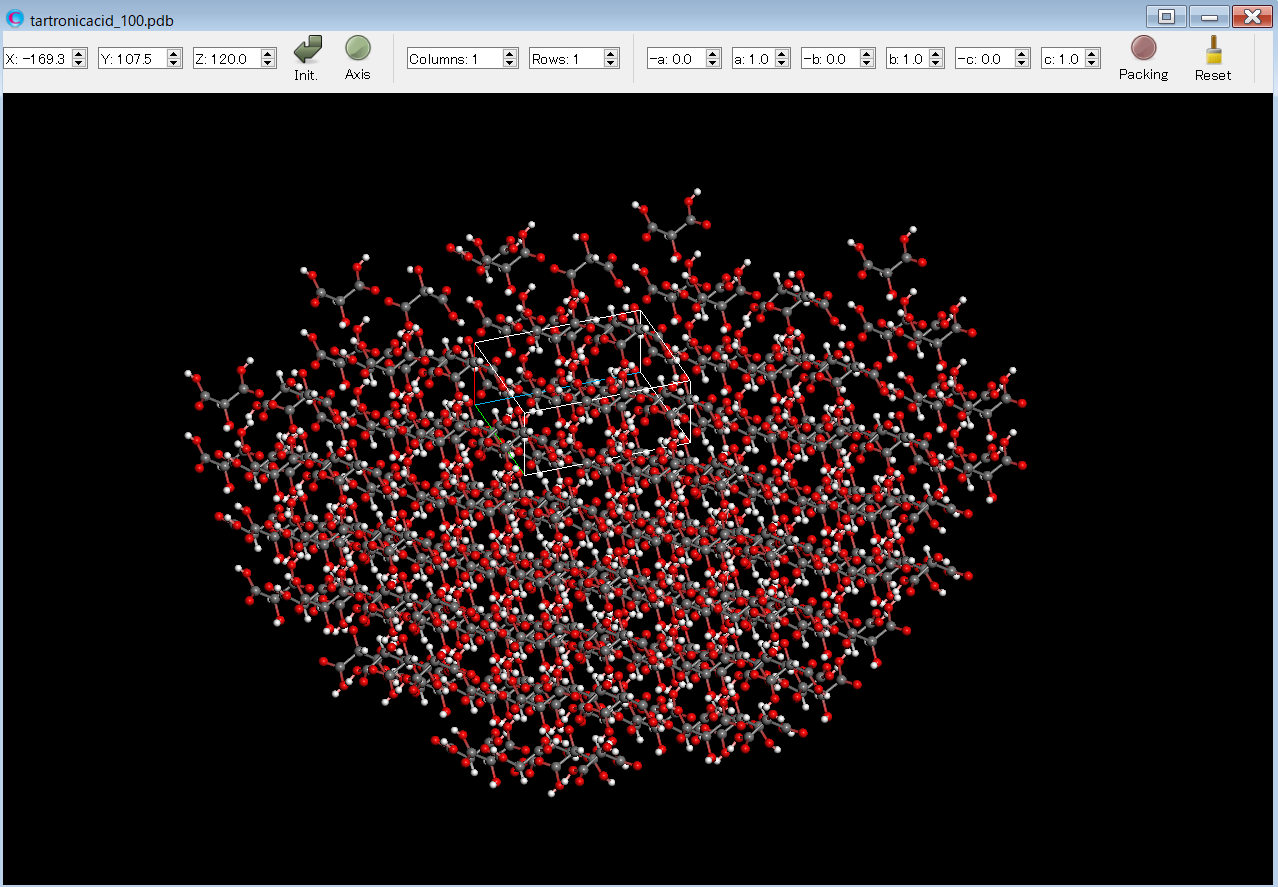The Emol of the target molecule is shown in the tartronicacid.bso file like below.

 * SUM OF INTERMOLECULAR INTERACTION ENERGIES OF MOLECULE
[IN] THE CRYSTAL PLANE

* CHANGE TO CUTOFF METHOD IN CALCULATION OF ELECTROSTATIC INTERACTIONS

ENERGY
H   K   L     (KCAL/MOL)
1   0   0     -10.7323

## [About the alphabet notation next to the energy]

Analysis of (010) plane of hydroxy malonic acid crystal is performed by using the tartronicacid.cmf file. Here, a crystal structure optimization is not performed.

The Emol of the target molecule is shown in the tartronicacid.bso file like below.

  * SUM OF INTERMOLECULAR INTERACTION ENERGIES OF MOLECULE
[IN] THE CRYSTAL PLANE

* CHANGE TO CUTOFF METHOD IN CALCULATION OF ELECTROSTATIC INTERACTIONS

ENERGY
H   K   L     (KCAL/MOL)
0   1   0     -39.2094  a
0   1   0     -38.4304  b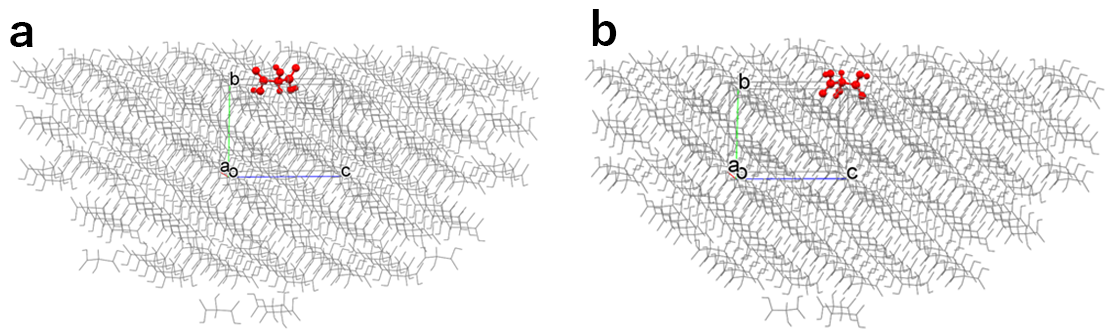The target molecule is automatically determined by the program. However, when there are some unique molecules in the specified crystal plane, the Emol with respect to each unique molecule are shown by distinguishing the results by the alphabet. The “a” and “b” are results of analysis with respect to the (010) plane, but the target molecule on the energy calculation is different.

## [Difference of “IN” and “ON” in “Molecular State:”]

In [Crystal Surface] tab in [Crystal Property] dialog, the state of the target molecule differs depending on whether [Molecular State:] is set to [IN] or [ON] as shown in the below figure.
In the execution by command line, it corresponds to whether to write [CRYSTAL_PLANE_STATE=IN] or [CRYSTAL_PLANE_STATE=ON] in the ini file.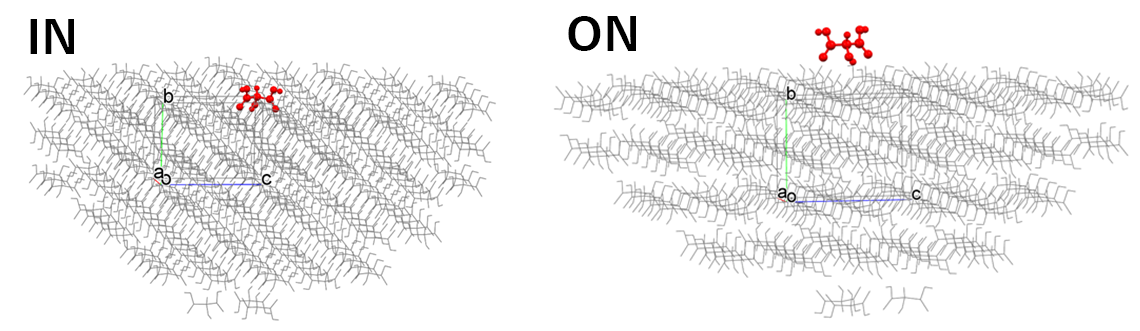[IN] selects the molecule composing of the specified crystal plane, and the Emol is calculated.
[ON] selects the molecule on the specified crystal plane, and the Emol is calculated.

## [How to use “Crystal Plane Translation”]

Analysis of (001) plane of hydroxy malonic acid crystal is performed by using the tartronicacid.cmf file. Here, a crystal structure optimization is not performed. CONFLEX builds a hemi-spherical crystal like the below figure and calculates Emol.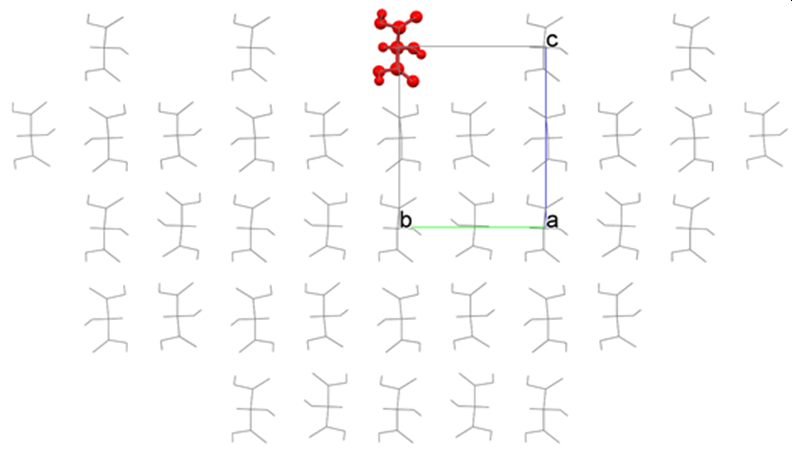Interface of the semi-spherical crystal is automatically determined by the program. However, you can change a position of the interface by setting value of [Crystal Plane Translation] in [Crystal Surface] tab on [Crystal Property] dialog. In the above figure, the position of the crystal plane is “0”, upper is positive direction and lower is negative direction.

For example, set the value of [Crystal plane Translation] to 1.0, and the interface moves to upper direction by 1.0 Ang..In the execution by command line, it corresponds to including [CRYSTAL_PLANE_TRANS=1.0] in the ini file.

tartronicacid.ini file

CRYSTAL
CRYSTAL_OPTIMIZATION=NONE
CRYSTAL_PLANE=(0,0,1)
CRYSTAL_PLANE_TRANS=1.0

As the result, a hemi-spherical crystal like the below figure is constructed, and the Emol is calculated.Comparing before and after moving the interface, we can see that the molecules indicated by the blue circle are included in the calculation of Emol due to the moving of interface. Please change the position of the interface if necessary.

## [How to use “Set Base Molecule”]

The target molecule is automatically determined by the program. However, you can change the target molecule by setting [Set Base Molecule] in [Crystal Surface] tab on [Crystal Property] dialog.
Here, by using the tartronicacid.cmf file as the input, an analysis of (100) plane of hydroxy malonic acid crystal will be explained as an example.

First, in order to get information of candidates for the target molecule, select [ON] in the pull-down menu of [Print Information of Candidates for Base Molecule:] in [Crystal Surface] tab, and a calculation is performed.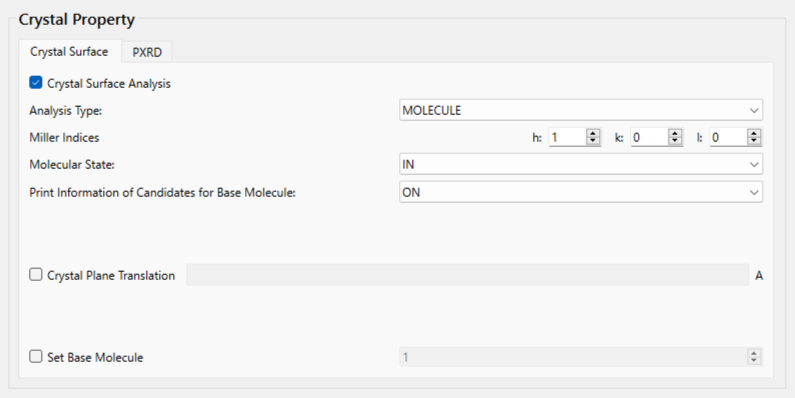In the case of an execution by command line, add [CRYSTAL_PLANE_PRINT=ON] to the ini file, and perform a calculation.

tartronicacid.ini file

CRYSTAL
CRYSTAL_OPTIMIZATION=NONE
CRYSTAL_PLANE=(1,0,0)
CRYSTAL_PLANE_PRINT=ON

In the tartronicacid.bso file, molecular index (IDX) and position of center of mass (POSITION) for the candidates are shown. In POSITION data, the crystal plane position is “0”, upper is positive direction, and lower is negative direction (see the below figure).

   IDX   POSITION (ANG.)
1      0.224367
2      0.224367
3     -0.224367
4     -0.224367
5      2.022633
6     -2.022633
7     -2.022633
8     -2.022633
9     -2.022633
10      2.471367

For example, in the case of selecting molecule with IDX=6, set “6” to [Set Base Molecule] of [Crystal Surface] tab.In the case of an execution by command line, add [CRYSTAL_PLANE_BASE=6] to the ini file, and perform a calculation.

tartronicacid.ini file

CRYSTAL
CRYSTAL_OPTIMIZATION=NONE
CRYSTAL_PLANE=(1,0,0)
CRYSTAL_PLANE_BASE=6

In the default setting, the hemi-spherical crystal model shown in the left figure is built, and the Emol is calculated. By setting “6” in [Set Base Molecule], the hemi-spherical crystal model shown in the right figure is built, and the Emol is calculated.In the case of the left figure, the Emol is -10.73 kcal/mol.
On the other hand, in the case of the right figure, the Emol is -34.15 kcal/mol.